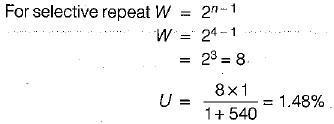Courses

# Test: Data Link Layer & MAC-Sublayer- 2

## 15 Questions MCQ Test GATE Computer Science Engineering(CSE) 2022 Mock Test Series | Test: Data Link Layer & MAC-Sublayer- 2

Description
This mock test of Test: Data Link Layer & MAC-Sublayer- 2 for Computer Science Engineering (CSE) helps you for every Computer Science Engineering (CSE) entrance exam. This contains 15 Multiple Choice Questions for Computer Science Engineering (CSE) Test: Data Link Layer & MAC-Sublayer- 2 (mcq) to study with solutions a complete question bank. The solved questions answers in this Test: Data Link Layer & MAC-Sublayer- 2 quiz give you a good mix of easy questions and tough questions. Computer Science Engineering (CSE) students definitely take this Test: Data Link Layer & MAC-Sublayer- 2 exercise for a better result in the exam. You can find other Test: Data Link Layer & MAC-Sublayer- 2 extra questions, long questions & short questions for Computer Science Engineering (CSE) on EduRev as well by searching above.
QUESTION: 1

### If an ethernet destination address is 07-01 -12 - 03 - 04 - 05, then that is a _____ address,

Solution:

07-01 - 12 - 03 - 04 - 05. The ethernet has more than one destinations but not all. So it is a multicast address not a broadcast address.

QUESTION: 2

### If N is the maximum sequence number, then the window sizes in selective reject and Go-Back-N protocols are respectively:

Solution:

Where n is maximum sequence number selective repeat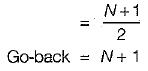QUESTION: 3

### CRC can detect all bursts of upto m errors, if generator polynomial G(x) is of degree

Solution:

CRC guarantees that all burst error of length equal to the degree of the polynomials are detected and also burst errors affecting an odd number of bits are detected.

QUESTION: 4

In a Go-back-N ARQ, if the window size is 63, what is the range of sequence numbers?

Solution:

In Go back-N maximum window size 2N- 1
And range of sequence member 0 to 2N- 1
So, 0 to 63.

QUESTION: 5

For stop and wait ARQ, for n data packets sent, ______________ acknowledgments are needed.

Solution:

It n data packets sent we need less than n data packet acknowledgments.

QUESTION: 6

In token ring, the tokens can be removed by

Solution:

In token ring N number of system choose 1 monitor. The responsibility of this monitor is
(i) Lost of Token,
(ii) Garbage frame,
(iii) Orphan frame.
If a station is disabled the token generated by it is removed from the token rina,

QUESTION: 7

Who strips the data frame from the token ring network?

Solution:

The station who sending data strips the data frame from the token ring network.

QUESTION: 8

Which of the following indicates the increasing order of accuracy in error detection?

Solution:

Single parity can detect single bit error, while block sum check can more than one bit error and CRC can detect all errors present in data.
So. sinale Daritv < Block sum check < CRC.

QUESTION: 9

A selective repeat ARQ is using 7 bits to represent the sequence numbers. What is the maximum size of the window?

Solution:

With selective repeat ARQ of 7 bits. Maximum window size = 27-1 - 26 = 64 Maximum sequence number = (64 -1) = 63.

QUESTION: 10

Which error detection method consists of a parity bit for each data unit as well as an entire data unit of parity bits?

Solution:

Two dimensional parity check consist parity bits for each data unit as well as an entire data unit of parity bits.

QUESTION: 11

The reference polynomial used in a CRC scheme  is x4 + x3 + 1. A data sequence 1010101010 is to be sent, Determine the actual bit string that is transmitted.

Solution:

Reference polynomial x4 + r3 + 1 =11001
Datasequence = 1010101010
On dividing 1010101010 by 11001,
We get CRC (i.e. remainder) = 0010
On appending this CRC on data sequence, we get the actual message transmitted i.e. 10101010100010.

QUESTION: 12

A 3000 km long trunk is used to transmit frames using a Go-Back-N protocol. The propagation speed is 6 jasec/ km and trunk data rate is 1.544 Mbps. We ignore the time taken to receive the bits in the acknowledgment. Frame size is 64 Bytes.

If Go-Back-N protocol is used, in order to achieve an efficiency of 100%, what is the maximum window size at the sender's side?

Solution:

For sliding window protocol with Go-Back-N
Data rate = 1.544 Mbps
Frame size = 64 bytes - 64 x 8 bits
= 512 bits
Propagation speed = 6x10-6 s/km Distance = 3000 km
Utilization = 100%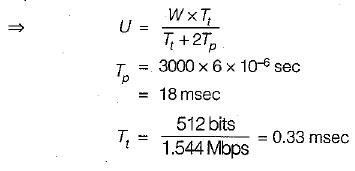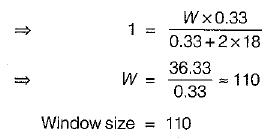QUESTION: 13

A 3000 km long trunk is used to transmit frames using a Go-Back-N protocol. The propagation speed is 6 jasec/ km and trunk data rate is 1.544 Mbps. We ignore the time taken to receive the bits in the acknowledgment. Frame size is 64 Bytes.

What is the maximum number of bits of the sequence number?

Solution:

Bits for sequence no. = 2no of bits > Window size
=>    W = 110
27 = 128 > 110
Hence 7 bits used for sequence number.

QUESTION: 14

A channel has bit rate of 1 Mbps and propagation delay of 270 msec. Frame size is of 125 bytes. Acknowledgment is always piggybacked onto data frames. Four bit sequence number is used, ignore header size.

What is the maximum achievable channel utilization for Go-Back-N?

Solution:

Data rate = 1 Mbps
Tp = 270 msec
F = 125 bytes
= 125 x 8 bits
= 1000 bits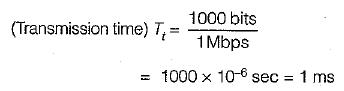Four bit sequence number
For Go-back-N, W= 16-1 = 15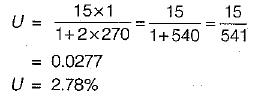QUESTION: 15

A channel has bit rate of 1 Mbps and propagation delay of 270 msec. Frame size is of 125 bytes. Acknowledgment is always piggybacked onto data frames. Four bit sequence number is used, ignore header size.

What is the maximum achievable channel utilization for Selective Repeat?

Solution: